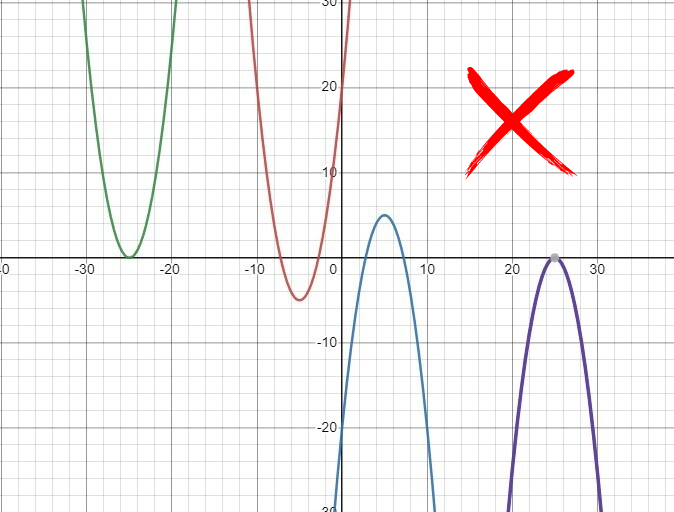# CAT 2022 Question Paper | Quant Slot 1

###### CAT Previous Year Paper | CAT Quant Questions | Question 5

CAT 2022 Quant was dominated by Arithmetic followed by Algebra. In Arithmetic, the questions were dominated by topics like Speed-time-distance, Mixture and Alligations. This year, there was a surprise. The questions from Geometry were relatively on the lower side as compared to the previous years. There were 8 TITA Qs this year. Overall this section was at a medium level of difficulty.

Question 5 : Let $a, b, c$ be non-zero real numbers such that $b^2 $lt 4 a c$, and $f$x)=a x^2+b x+c$. If the set $S$ consists of al integers $m$ such that $f(m)$lt0$, then the set $S$ must necessarily be 1. the set of all integers 2. either the empty set or the set of all integers 3. the empty set 4. the set of all positive integers ## 🎉Get Upto ₹10,000 off on our CAT 23/24 courses.🎉Get flat ₹6,000 off on IPM courses.Valid till 30th September 2023! #### ★Register Now! ### Video Explanation ## Best CAT Coaching in Chennai #### CAT Coaching in Chennai - CAT 2022Limited Seats Available - Register Now! ### Explanatory Answer We are given the definition of the function, $f$x)=a x^2+b x+c$, and been told that $b^2<4 a c$. This means that f(x) has imaginary roots and therefore no real roots at all.
If a function has no real roots, the graph of the function can never touch the x-axis, because touching the x-axis means, for some real value x, the value of f(x) is 0. In other words, it means that the function has roots for some real value x.When we graph this function quadratic function f(x), we get a parabola, that should never touch the x-axis. Such a parabola should be completely above the x-axis or completely below the x-axis.If it is completely above the x-axis:
It means that the value of f(x) is always positive for any value of x.
The set of values of x that satisfies the condition that f(x) < 0 is an empty set.
If it is completely below the x-axis:
It means that the value of f(x) is always negative for any value of x.
The set of values of x that satisfies the condition that f(x) < 0 is the set of all real numbers.
Since set S contains all the integers ‘m’ that satisfy the above conditions, Set S is either an empty set or the set of all integers.

##### The answer is 'either the empty set or the set of all integers'

Choice B is the correct answer.

###### CAT Coaching in ChennaiCAT 2023

Classroom Batches Starting Now! @Gopalapuram

###### Best CAT Coaching in Chennai Introductory offer of 5000/-

Attend a Demo Class

##### Where is 2IIM located?

2IIM Online CAT Coaching
A Fermat Education Initiative,
58/16, Indira Gandhi Street,
Kaveri Rangan Nagar, Saligramam, Chennai 600 093

##### How to reach 2IIM?

Mobile: (91) 99626 48484 / 94459 38484
WhatsApp: WhatsApp Now
Email: info@2iim.com